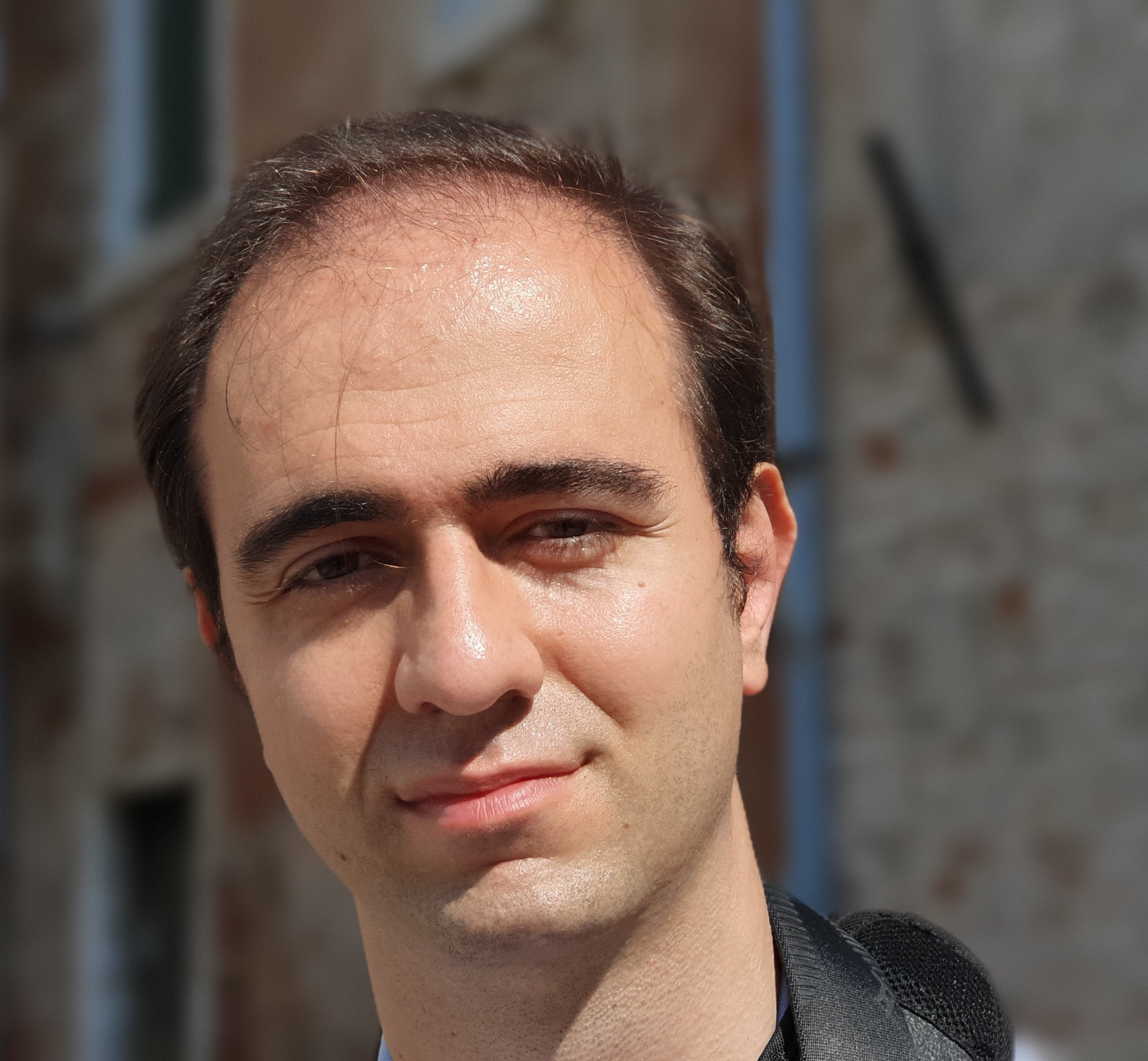Researcher at INFN Laboratori Nazionali di Frascati

## The Higgs transverse momentum distribution in gluon fusion as a multiscale problem

Emanuele Bagnaschi (DESY) and Alessandro Vicini (INFN, Milan and Milan U.)

JHEP 01 (2016) 056

e-print: 1505.00735 [hep-ph]

We consider Higgs production in gluon fusion and in particular the prediction of the Higgs transverse momentum distribution. We discuss the ambiguities affecting the matching procedure between fixed order matrix elements and the resummation to all orders of the terms enhanced by $\log(p_{T}^{H}/m_{H}​)$ factors. Following a recent proposal, we argue that the gluon fusion process, computed considering two active quark flavors, is a multiscale problem from the point of view of the resummation of the collinear singular terms. We perform an analysis at parton level of the collinear behavior of the $\mathcal{O}\left({\alpha}_s\right)$ real emission amplitudes, relying on the collinear singularities structure of the latter, we derive an upper limit to the range of transverse momenta where the collinear approximation is valid. This scale is then used as the value of the resummation scale in the analytic resummation framework or as the value of the h parameter in the ${\tt POWHEG-BOX}$ code. A variation of this scale can be used to generate an uncertainty band associated to the matching procedure. Finally, we provide a phenomenological analysis in the Standard Model, in the Two Higgs Doublet Model and in the Minimal Supersymmetric Standard Model. In the two latter cases, we provide an ansatz for the central value of the matching parameters not only for a Standard Model-like Higgs boson, but also for heavy scalars and in scenarios where the bottom quark may play the dominant role.